#### You may also like### Exploring Wild & Wonderful Number Patterns

EWWNP means Exploring Wild and Wonderful Number Patterns Created by Yourself! Investigate what happens if we create number patterns using some simple rules.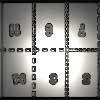### Magazines

Let's suppose that you are going to have a magazine which has 16 pages of A5 size. Can you find some different ways to make these pages? Investigate the pattern for each if you number the pages.### Pebbles

Place four pebbles on the sand in the form of a square. Keep adding as few pebbles as necessary to double the area. How many extra pebbles are added each time?

# Tables Without Tens

## Tables Without Tens

You will need a piece of squared paper for this activity. If you have none you can get a sheet here.
Write the ones digits of the numbers in the two times table from $1 \times 2$ up to $10 \times 2$ in a line. (Leave some room at the top of the paper, and some space to the left and right.)
It should look like this: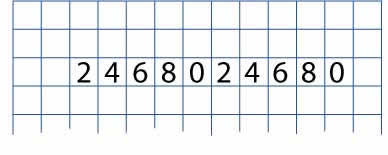You might be able to see some patterns in these numbers.
Now, do the same thing with the multiples of three. Remember, just write the ones digits, this time directly underneath the line of the two times table, like this: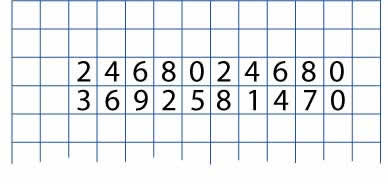Continue by writing in the four and five times tables in the same way. Again, just using the ones digits.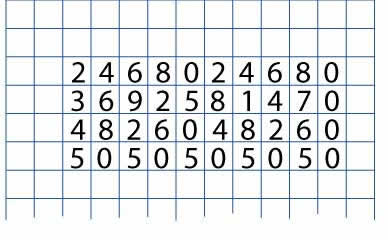Now look at the whole array of numbers you have created.
What patterns can you find?
Try to explain why the patterns occur.
What do you notice about these four sets of numbers?
Can you predict what would happen next if we wrote in the next times table?

***************

Well, why not add in the tables of sixes, sevens, eights, nines and finally tens?
After that, for the sake of completeness, we could put in the table of ones and zeros.
Do you have a grid that looks like this?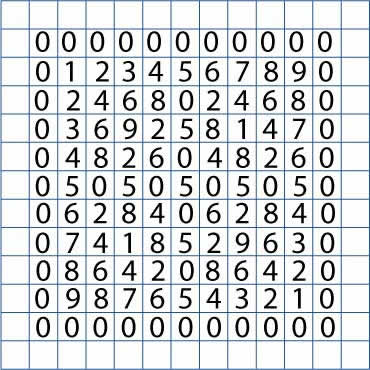What patterns are there here?
Can you predict what you will find?
How might you record the repeats that you find?
Each line could be written like this: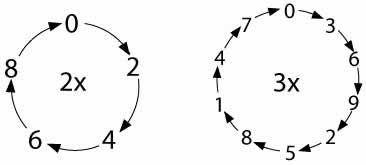But you will probably find some other ways which are just as good.
You could try writing all the tables like that.
Are some tables the same or similar to others?
Does it matter which way the arrows go?
What can you discover about the pattern of repeats?
Can you predict what you will find out about 'pairs' of tables?

### Why do this problem?

This problem involves learners in making and proving conjectures using patterned numbers and arithmetic sequences. It is, incidentally, a very interesting way of revising multiplication tables! It is also very useful for getting learners to predict what they think they will find out.

This is a good class investigation in that it can be taken on a long way but patterns can be found at an early stage so those who work more slowly will be doing some discovering.

### Possible approach

You could introduce the investigation as suggested or, alternatively, from a standard $10 \times 10$ 'table-square' simply dropping the tens. However, this does remove some of the exploration and discovery.

After the initial introduction learners could work in pairs so that they are able to talk through their ideas with a partner. Plenty of squared and plain paper should be available. Squared paper can be found here.

You could show the group the cycles of repeats which are a very useful way of recording but learners may find another better way! When making them, make sure that the arrows are put in because this matters.
They can be put in like this: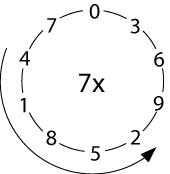At the end of the lesson the group should come together to discuss their explorations and discoveries. The factors of $10$ and the complements in $10$ (the numbers that add to make $10$) should arise in interesting ways. When pressed, can they give satisfactory explanations?

### Key questions

What do you think you will find out from doing this?
How often does it repeat?
Which digits are there in the line?
Have you looked along the rows and up and down the columns?
Have you looked at any of the diagonals?
Would it help, when you're finding repeats, to extend the rows to $11$ lots of the number, $12$ lots, $13$ lots?
How did you make each row in the first place?

### Possible extension

After exploring the many patterns in the investigation given learners could try Diagonal Sums.
Some ideas for more extensions can be found on this sheet: wordpdf.

### Possible support

Suggest using a standard $10 \times 10$ 'table-square' to help with the tables. If even this is proving difficult, start by using a $10 \times 10$ 'table-square' [such as this one] that can be written on and crossing out the tens figures. The resulting ones-numbers can then be transferred to a plain sheet of squared paper. This ready-made sheet might help.